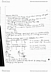# ECON 1000 Lecture Notes - Bounded Rationality, Neuroeconomics, Marginal Utility

97 views2 pagescastroariane563 and 39059 others unlocked10
Verified Note
10 documents

## Document Summary

The utility-maximizing choice: we can find the utility-maximizing choice by looking at the total utility that arises from each affordable combination, the utility-maximizing combination is called a consumer equilibrium. A consumer"s total utility is maximized by following the rule: Spend all available income: equalize the marginal utility per dollar for all goods. The marginal utility per dollar is the marginal utility from a good divided by its price. Total utility is maximized when good a and good b have the same marginal utility per dollar. If mua/pa > mub/pb, then mua decrease and mub increase. If mua/pa < mub/pb, then mua increase and mub decrease. A fall in the price of a movie: when the price of a good falls the quantity demanded of that good increases the demand curve slopes downward. A rise in the price of pop: we know that mup/pp falls, so before the consumer changes the quantities bought,